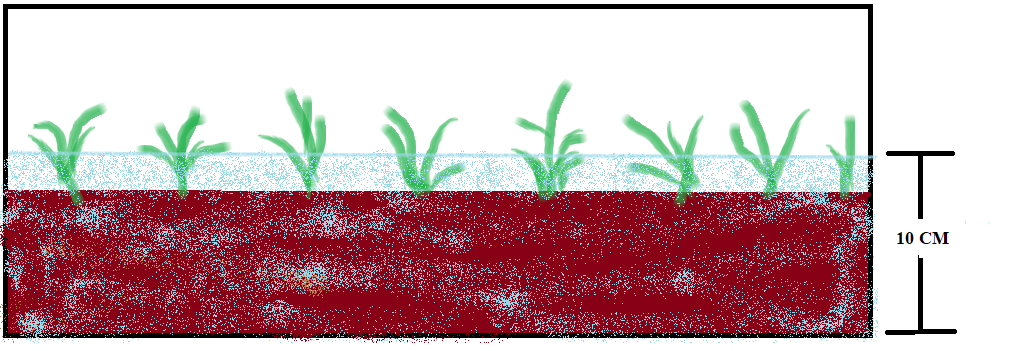### Irrigation Engineering

Things To Know

1. Crop period: Time period between sowing to harvesting.

2. Base period : Time period between first watering to last watering before harvesting.

1.

DELTA (D)

2. DUTY (D)

1. DELTA  (D)
Definition: Total depth of water required for the crop to mature
To understand the concept, let's take an example of a crop X which has a base period of 100 days and needs a water level of 10cm as in the picture below. Due to evaporation or by absorption of water we have to irrigate the crop once in 10 days.So how many cm water is required for thePlant to grow?
Base period (B)=100 days
Frequency (F)=10 days
Amount of water needed=10cm
No. of times to be irrigated = Base period/Frequency => 100/10
=10times.
= no. of irrigated time X  frequency => 10 X 10= 100cm.

1. DUTY (D)
Definition: Area to be irritated with unit volume of water.
To understand this, we have a crop which need to be watered for 1m depth also we have a tank of water which contains 100 m^3 of water with this how much area can be irritated?
V= B X V X H=100m^3.
V/D =Bx